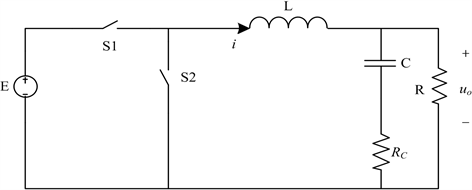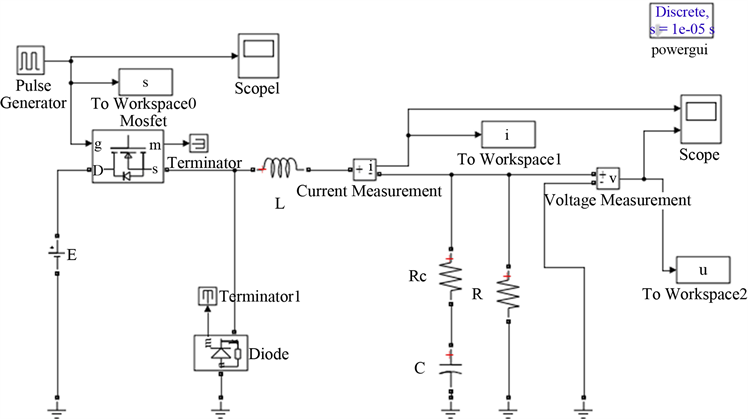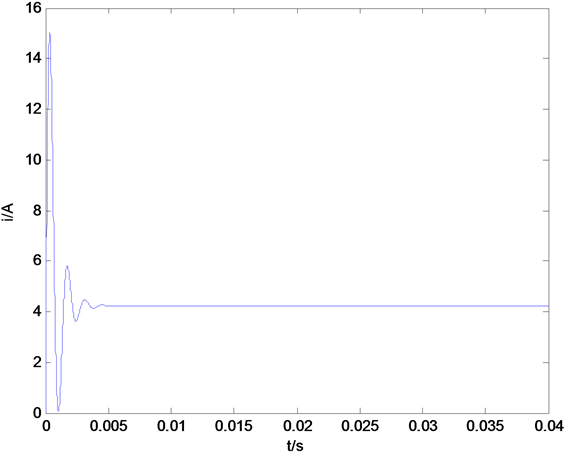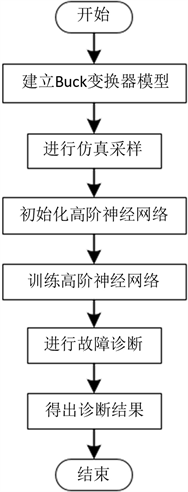# 基于高阶神经网络的电力变换器滤波故障诊断方法设计Design of Filtering Fault Diagnosis Method for Power Converter Based on High-Order Neural Network

DOI: 10.12677/AIRR.2019.82011, PDF, HTML, XML, 下载: 452  浏览: 714

Abstract: A fault diagnosis method for power converter based on high-order neural network algorithm is proposed. Taking the fault diagnosis in Buck converter as an example, a diagnostic structure for high-order neural network is designed. And taking the voltage and current values of Buck converter at different working conditions under the condition of continuous current as samples, the high-order neural network is trained to realize the fault diagnosis of Buck converter.

1. 引言

2. 模型建立Figure 1. The equivalent schematic diagram of the Buck converterFigure 2. The Simulink model of the Buck converterTable 1. The parameters of the Buck converterFigure 3. The inductance current curve of the Buck converterFigure 4. The output voltage curve of the Buck converter

3. 基于高阶神经网络的故障诊断Table 2. The correspondence between the operating condition and the HONN output

${h}_{j}\left(k\right)=f\left(\underset{i=1}{\overset{2}{\sum }}{w}_{ji}\left(k\right){x}_{i}\left(k\right)\right)$ (1)Figure 5. The structure chart of the HONN

${y}_{M}\left(k\right)=g\left(\underset{j=1}{\overset{2}{\prod }}{\xi }_{Mj}\left(k\right){h}_{j}\left(k\right)\right)$ (2)

${y}_{M}\left(k\right)=g\left(\underset{j=1}{\overset{2}{\prod }}{\xi }_{Mj}\left(k\right)f\left(\underset{i=1}{\overset{2}{\sum }}{w}_{ji}\left(k\right){x}_{i}\left(k\right)\right)\right)$ (3)

${w}_{ji}\left(k+1\right)={w}_{ji}\left(k\right)-\eta \frac{\partial E\left(k\right)}{\partial {w}_{ji}\left(k\right)}$ (4)

${\xi }_{Mj}\left(k+1\right)={\xi }_{Mj}\left(k\right)-\eta \frac{\partial E\left(k\right)}{\partial {\xi }_{Mj}\left(k\right)}$ (5)

$E\left(k\right)=\frac{1}{2}\underset{M=1}{\overset{3}{\sum }}{\left({\stackrel{¯}{y}}_{M}\left(k\right)-{y}_{M}\left(k\right)\right)}^{2}$ (6)Figure 6. The fault diagnosis flow chart of the HONN

4. 结论

  任磊, 龚春英. 一种电力电子变换器功率MOSFET阈值电压在线监测方法[J]. 电工技术学报, 2018(15): 3627-3634.  丁世宏, 王加典, 黄振跃, 等. Buck变换器扰动补偿控制算法及实现[J]. 农业工程学报, 2015, 31(8): 214-220.  徐帅, 杨欢, 王田刚, 等. 电力电子变换器可靠性研究[J]. 北京交通大学学报, 2015, 39(5): 125-132.  朱旭东, 戴文战, 郎燕峰. 基于神经网络的方法在故障诊断中的应用[J]. 机电工程, 2003, 20(5): 75-78.  寇琳琳. 基于高阶神经网络的遥感图像分类识别研究[J]. 电大理工, 2008(3): 3-5.  余发山, 康洪. 基于GA优化BP神经网络的液压钻机故障诊断[J]. 电子测量技术, 2016, 39(2): 134-137.